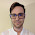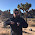Wednesday, May 13, 2015

Solow production function and nominal values

Editor's note: Still cleaning out my backlog of unfinished posts. This is a question I've had and is addressed to the economists out there ... even after reading through a couple macro textbooks, I still don't have a good answer. File under questioning whether real and nominal are actually useful concepts in economics.

As noted here, the Solow growth model Cobb-Douglas production function

$$Y(t) = A(t) K(t)^{\alpha} \; L(t)^{\beta}$$

works really well if $A = constant$, and $\beta \neq 1 - \alpha$ and both $K$ and $Y$ represent nominal values.

One thing I have not been able to understand is that when using real values in the traditional version with $\alpha + \beta = 1$, call them $Y = P(t) y(t)$ and $K = P(t) k(t)$, you end up with a factor of the price level relative to the nominal version ...

$$y(t) = A(t) k(t)^{\alpha} \; L(t)^{1 - \alpha}$$

$$P(t) y(t) = A(t) P(t) k(t)^{\alpha} \; L(t)^{1 - \alpha}$$

$$Y(t) = A(t) P(t)^{1 - \alpha} K(t)^{\alpha} \; L(t)^{1 - \alpha}$$

The extra factor of $P^{1- \alpha}$ could be absorbed by the $L$, but labor is given in terms of hours or people (or 'labor units'), not wages ... or is it?

The nominal version of the equation seems to make more sense (it is a combinatorial problem with dimensionless nominal dollar units and 'nominal' labor units).

The real version leads to the mystery of the fall-off in Total Factor Productivity (the 'great stagnation'), and TFP -- i.e. phlogiston -- being the majority of economic growth.
Editor's note, added 5/13/2015: Sounds like a no-brainer, but it requires re-thinking all the other instances of real vs nominal ... as I've addressed here. This post was an initial draft for the one at that link that I abandoned and restarted.
Real and nominal may actually be an attempt by economists to adjust for entropy terms

1.If you're concerned with the majority of "real" economic growth being caused by changes in TFP, I suggest you look into endogenous growth theory: https://en.wikipedia.org/wiki/Endogenous_growth_theory

Also, I believe FRED's measure of Canada's TFP has been pretty stagnant since 1950, so that may be worth looking into.

1.Hi John, thanks for the pointers ... the issue I'm having is more general than a specific model -- the Solow production function just illustrates it pretty well.

If you use nominal values there is no mystery, 'TFP' is just a constant, and the model is pretty empirically accurate. If you use real values, there is the mathematical weirdness above (being unable to convert from real to nominal values in the formula), the model isn't very accurate, and TFP is this major factor accounts for most of economic growth. As I said in the closing it seems to be a no-brainer ...

2.The extra factor can be absorbed by labor, or at least it can be absorbed with no interpretative problems. The Cobb-Douglas production function is mostly used because it spits out expressions like " w L = (1 - a) Y ", i.e. labor's real share of real GDP is constant. "Reflating" real product by multiplying it by P changes that equation to " P w L = (1 - a) P Y ", i.e. labor's nominal share of nominal GDP is constant, which is the same thing as before, but multiplied by a price index. That real demand for labor doesn't change with prices is a good thing (in economics).

1.Thanks for the description -- that seems to make sense mathmatically.

I also realized that I make a mistake; since the equation follows from

$$\frac{\partial Y}{\partial K} = \alpha \; \frac{Y}{K}$$

any transformation of the form $Y \rightarrow c(t) Y$, $K \rightarrow c(t) K$ will leave the result unchanged (homogeneity of degree zero). That is that there shouldn't be a factor left over -- I'm really looking at the equation:

$$\frac{Y}{Y_{0}} = \lambda \left( \frac{K}{K_{0}}\right)^{\alpha} \left( \frac{L}{L_{0}}\right)^{\beta}$$

and so have to change the $K_{0}$ too. No need to absorb it into $L$.

Comments are welcome. Please see the Moderation and comment policy.

Also, try to avoid the use of dollar signs as they interfere with my setup of mathjax. I left it set up that way because I think this is funny for an economics blog. You can use € or £ instead.